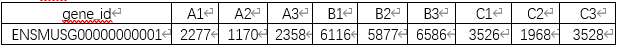### ANOVA/Kruskal-Wallis

Function: Multiple groups of inspection tools. Kruskal-Wallis test and ANOVA test can be used to conduct difference analysis for multiple groups of independent samples. At the same time, the result of post hos multiple comparisons can be output to obtain the difference mainly come from.

File：The variable file to verify

Gruop: Defines the sample grouping information file

Drawing type：User can choose the corresponding graphics according to the needs of the use situation. Boxplot, violin chart, bar chart are available at present.

Inspection methods: ANOVA, also know as"F test". It can used to test the significance of the difference between the mean of two or more samples. If significant differences are found between samples, the Tukey HSD can be selected to find out which comparison group the differences.

Kruskal-Wallis rank sum test is a non-parametric test, which can replace the sample value with the sample rank for the difference analusis of multiple independent samples with difference sample size. After the difference analysis of multiple groups of samples, it is found that there are differences among mutiple groups of samples, then Nemenyi test can be further used to conduct multiple comparison between groups, and the significance analysis of parirings of differences among multiple samples can be carried out to determine which comparison group the differences.

Post Hos Multiple Comparisons: Post Hos Multiple Comparisons is used to determine the source of the discrepancy. When there were differences between multiple groups, pairwise comparison was conducted to determine whether there was any difference between the two groups. Genedenovo provides two testing methods: Tukey HSD inspection and Nemenyi inspection.

Significant differences between groups were shown: Postmortem results can be displayed using P values, asterisks(*), and letters. If the P value is displayed, the specific P value is output directly in the graph. If displayedwith "*", "*" means P<0.05, and the difference is significant. "**" means P<0.01, the difference is extermely significant. No label indicates no significant difference. If presented in the form of letters, the same letters in the two groups indicated no significant difference, while the same letters in the two groups indicated significant difference.

Output: Output compressed package files, including table files and graphics files(pdf format, png format),the rest of the files for OS platform source files.1) Table files2) Figure files

Box-plotFigure interpretation: Box-plot can be used to show the distribution of data within and between groups. The abscissa represents the group, and the ordinate represents the data distance within the group. In the box diagram: the maximum value of the effective data distribution(the top of the line), the minimum value of the sffective data distribution (the bottom of the line), the  median(the black dot), the upper quartile(the top border of the rectangle), the lower quartile(the bottom border of the rectangle), and the invalid data(the outlier of the line). The difference between groups can be shown by P value, star symbol and letter symbol.

Violin diagramViolin diagram is a combination of box plot and kernel density diagram, used to show data distribution and its probability density, mainly showing the distribution of data. The 'width' of the graph represents the number of data in this range.

Bar diagramInput: The file must be in 'txt' format. User can optionally open the data in Excel and save it as a '(*.txt)'

1) File: ( Example is qRT-PCR results). Name of behavior sample in the first column, name of differencr test variable in the first column. There are at least 3 duplicate samples in each group.2) Group:

Defines grouping information that describes the grouping of samples in a difference test file.Post Hos Multiple Comparisons: Nemenyi inspection

Significant differences between groups were shown:  P

Output:

1) Table files2) Figure files